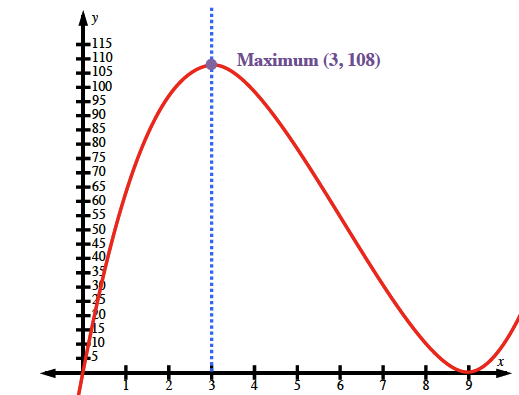### Home > PC > Chapter 7 > Lesson 7.2.3 > Problem7-66

7-66.

The sum of two positive numbers is $9$. Find the maximum product that will result from the first number and the square of the second number. Find an expression that can be graphed to find the maximum value. Then use your graphing calculator to find the maximum product.

Let $x =$ 1st number
Let $y =$ 2nd number
Let $P =$ product of the numbers

$x + y = 9$
$P = xy ^{2}$

$P = x\left(9 − x\right)^{2}$